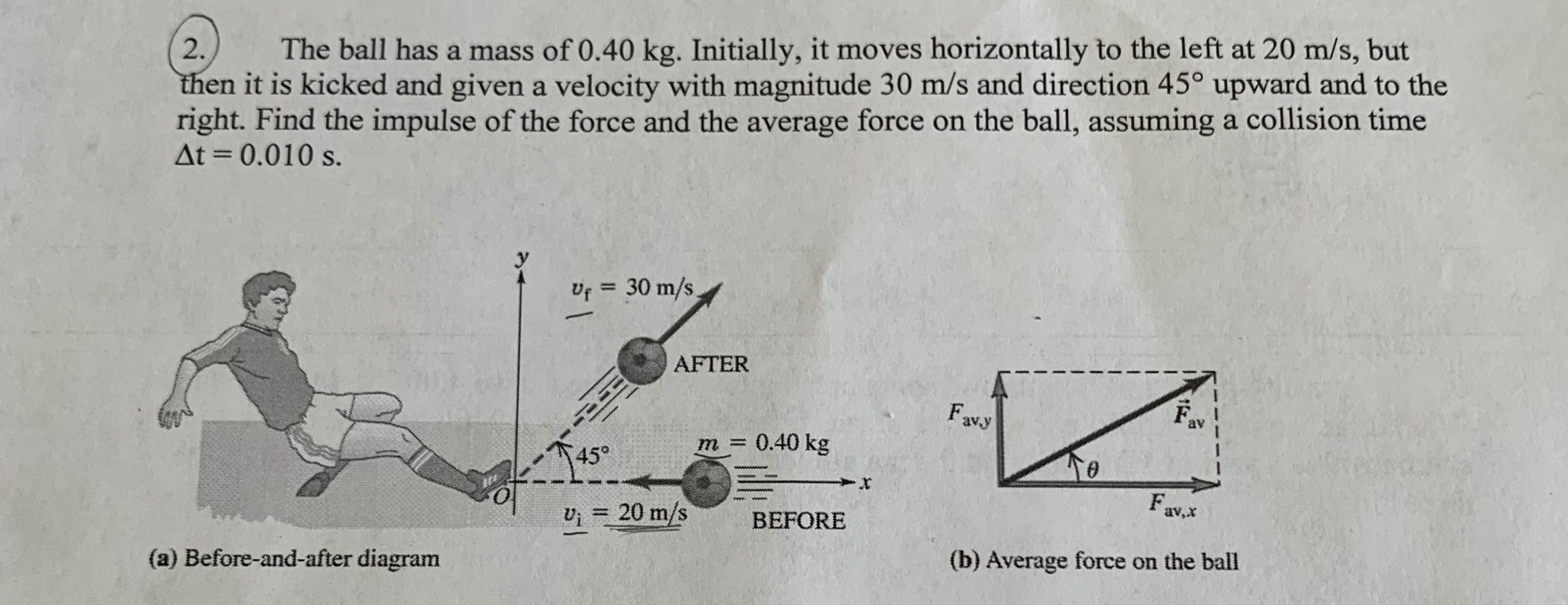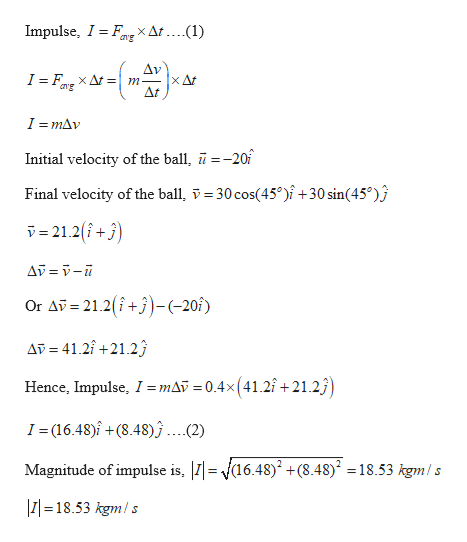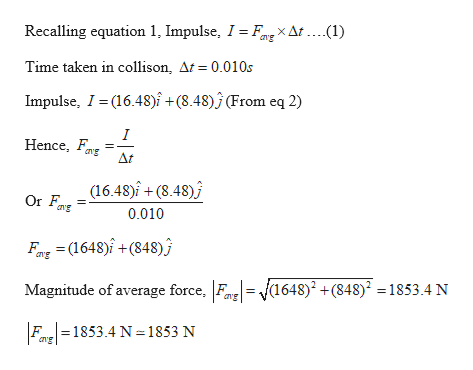# The ball has a mass of 0.40 kg. Initially, it moves horizontally to the left at 20 m/s, but2.then it is kicked and given a velocity with magnitude 30 m/s and direction 45° upward and to theright. Find the impulse of the force and the average force on the ball, assuming a collision timeAt 0.010 s.Uf 30 m/sAFTERavy0.40 kgm.45°wwwwwF.Ui 20 m/sav,xBEFORE(a) Before-and-after diagram(b) Average force on the ball

Question
54 viewshelp_outlineImage TranscriptioncloseThe ball has a mass of 0.40 kg. Initially, it moves horizontally to the left at 20 m/s, but 2. then it is kicked and given a velocity with magnitude 30 m/s and direction 45° upward and to the right. Find the impulse of the force and the average force on the ball, assuming a collision time At 0.010 s. Uf 30 m/s AFTER avy 0.40 kg m. 45° wwwww F. Ui 20 m/s av,x BEFORE (a) Before-and-after diagram (b) Average force on the ball fullscreen
check_circle

Step 1

Given:

Mass of the ball, m = 0.4 kg

Initial velocity = 20 m/s horizontally left

Final velocity = 30 m/s at 45 degrees from horizontal

Time of collision = 0.010 s

Step 2

Calculating the impulse during the collision:help_outlineImage TranscriptioncloseImpulse, I FE XAt ....(1) cng Av x Д At I F XAt спg I = mAv Initial velocity of the ball, ū=-20 Final velocity of the ball, v30 cos(45°)f+30 sin(45°) v= 21.2(+) Or Av 21.2(+)-(-20f) AD 41.2+21.2 Hence, Impulse, I = mAỹ = 0.4x(41.2î+21.2 I =(16.48)i+(8.48) ...(2) Magnitude of impulse is, (16.48) +(8.48) =18.53 kgm/s 2 |I 18.53 kgm fullscreen
Step 3

Calculating the average for...help_outlineImage TranscriptioncloseRecalling equation 1, Impulse, I = Fng X At . ...(1) Time taken in collison, At = 0.010s Impulse, I(16.48)î +(8.48)j (From eq 2) I Hence, ang At (16.48)î +(8.48) ang 0.010 Fax(1648)+(848) g 1648)2 +(848)2 =1853.4 N Magnitude of average force, Fn Fa1853.4 N 1853 N fullscreen

### Want to see the full answer?

See Solution

#### Want to see this answer and more?

Solutions are written by subject experts who are available 24/7. Questions are typically answered within 1 hour.*

See Solution
*Response times may vary by subject and question.
Tagged in

### Collisions